# Team:HUST-China/Modelling/DDE Model

### DDE MODEL

We had to validate the feasibility of our supposed genetic pathway in the first place. We wanted to know if our engineered cells could generate periodical signal, if it's stable against environment changes, and how its period can be adjusted. To solve these issues, we build our model for single cell using DDEs model.

#### Methods

1. Establish DDEs based on mass action law;
2. Discuss DDEs' stability and sensitivity through examining their characteristic equations;
3. Determine the most effective factors in changing period.

#### Assumptions

1.Only AraC or LacI protein can bind to hybrid promoter in the same time.

#### Establishing DDEs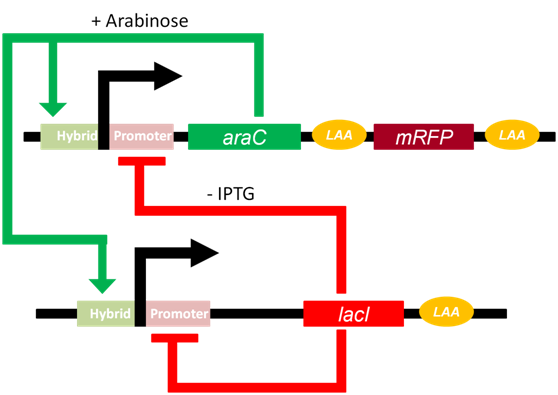Fig 1.The pathway of genetic oscillator

The Arabinose Operon and the lac Operon is the core to the functioning of the oscillator. With the presence of Arabinose, dimeric AraC can induce the expression of downstream gene; On the other hand, with minor presence of IPTG, tetrameric LacI may suppress the expression of downstream gene. However, AraC and LacI cannot combined with promoters simultaneously. Therefore, we have: $$D+2a\overset{k_1}{\underset{k_{-1}}\rightleftharpoons} D_1$$ $$D+4r\overset{k_2}{\underset{k_{-2}}\rightleftharpoons} D_2$$ where $D$ stands for ratio of promoters which don't combine with any protein among all promoters, a for AraC protein(activator), r for LacI protein(repressor), $D_1$ for ratio of operons combined with AraC dimer, $D_2$ for ratio of operons combined with tetrameric LacI, $k_1$,$_{-1}$,$k_2$ and $k_{-2}$ are reaction rate constants. We assumed that comparing with numbers of operons, that of protein is significantly large enough to ignore whose changes brought by combination of protein and operons. According to law of mass action, we had: $$\frac{dD}{dt}=-k_1Da^2+k_{-1}D_1-k_2Dr^4+k_{-2}D_2$$ $$\frac{dD_1}{dt}=k_1Da^2-k_{-1}D_1$$ $$\frac{dD_2}{dt}=k_2Dr^4-k_{-2}D_2$$ With $a,a_0,r,r_0 > 0$, we found that eigenvalues $\lambda < 0$. Thus, when $t \rightarrow \infty$ we had$\frac{dD}{dt}=\frac{dD_1}{dt}=\frac{dD_2}{dt} = 0$. We also have $D+D_1+D_2 = 1$, so we arrive at: $$D=\dfrac{k_{-2}}{k_2r^4+k_{-2}+\frac{k_1k_{-2}a^2}{k_{-1}}}$$ $$D_1 = \frac{k_1a^2}{k_{-1}}D$$ $$D_2 = \frac{k_2r^4}{k_{-2}}D$$ Denote $a_0 = \frac{k_{-1}}{k_1}$ and $r_0 = \frac{k_{-2}}{k_2}$, we had: $$D = \dfrac{1}{1+\frac{a^2}{a_0}+\frac{r^4}{r_0}}$$ $$D_1 = \dfrac{a^2}{a_0(1+\frac{a^2}{a_0}+\frac{r^4}{r_0})}$$ $$D_2 = \dfrac{r^4}{r_0(1+\frac{a^2}{a_0}+\frac{r^4}{r_0})}$$ What's worth mentioning is that both $a_0$ and $r_0$ are constant that are related to IPTG(mM) and Arabinose(%). $$a_0 = \dfrac{(6.25+ara^2)(1+\frac{iptg^2}{3.24})}{100ara^2}$$ $$r_0 = \dfrac{1}{2000000(\dfrac{0.19}{1+{\frac{iptg}{0.035}}^2+0.01})}$$ During the transcription process, we had: $$D_1\xrightarrow{k_3}R_{a/r}$$ $$D_2\xrightarrow{k_4}R_{a/r}$$ $$D\xrightarrow{k_5}R_{a/r}$$ where $R_{a/r}$denotes mRNAs of either AraC or LacI. $k_3$, $k_4$ and $k_5$ are transcriptional reaction rate constants. For convenience, we assumed that transcription rate for either AraC or LacI are exactly the same. During the translation and folding processes, we have: $$R_a\xrightarrow{t_a}a_{uf}$$ $$R_r\xrightarrow{t_r}r_{uf}$$ $$a_{uf}\xrightarrow{k_{fa}}a$$ $$r_{uf}\xrightarrow{k_{fr}}r$$ where $a_{uf}$ and $r_{uf}$ are unfolded proteins, $t_a$ and $t_r$ are translational reaction rate constants while $k_{fa}$,$k_{fr}$ are folding rates constants. In degradation process, we had: $$R_{a/r}\xrightarrow{d_{a/r}}\varnothing$$ $$a_{uf}\xrightarrow{\lambda f(x)}\varnothing$$ $$r_{uf}\xrightarrow{f(x)}\varnothing$$ $$a\xrightarrow{\lambda f(x)}\varnothing$$ $$r\xrightarrow{f(x)} \varnothing$$ where $d_{a/r}$, $f(x)$ and $\lambda f(x)$ are degradation rate constants.
According to law of mass reaction, we had: $$\frac{dR_a}{dt} = copy_a(k_3D_1+k_4D_2+k_5D)-d_{a/r}R_a$$ $$\frac{dR_r}{dt} = copy_r(k_3D_1+k_4D_2+k_5D)-d_{a/r}R_r$$ $$\frac{da_{uf}}{dt} = t_aR_a-k_{fa}a_{uf} - \lambda f(x)a_{uf}$$ $$\frac{dr_{uf}}{dt} = t_rR_r-k_{fr}r_{uf} - f(x)r_{uf}$$ $$\frac{da}{dt} = k_{fa}a_{uf} - \lambda f(x)a$$ $$\frac{dr}{dt} = k_{fr}r_{uf} - f(x)r$$ where $copy_a$ and $copy_r$ are plasmid copies that are transfected into E.coli.
Transcriptional and translational processes of genes take time and consequently, proteins AraC and LacI that combined to promoters can be seen as those started transcription process before a specific time interval $\tau _1$ and $\tau _2$. Thus we attach delay to three parameters: $$D = \dfrac{1}{1+\frac{a(t-\tau _1)^2}{a_0}+\frac{r(t-\tau _2)^4}{r_0}}$$ $$D_1 = \dfrac{a(t-\tau _1)^2}{a_0(1+\frac{a(t-\tau _1)^2}{a_0}+\frac{r(t-\tau _2)^4}{r_0})}$$ $$D_2 = \dfrac{r(t-\tau _2)^4}{r_0(1+\frac{a(t-\tau _1)^2}{a_0}+\frac{r(t-\tau _2)^4}{r_0})}$$
Table 1-1 Parameters and variables used in DDEs
Parameters and Variables Value Unit
$a_0$ $\dfrac{(6.25+ara^2)(1+\frac{IPTG^2}{3.24})}{100ara^2}$
$r_0$ $\dfrac{1}{2000000\frac{0.19}{1.0+(\frac{IPTG}{0.035})^2+0.01}}$
$D$ $\dfrac{1}{1+\frac{a^2}{a_0}+\frac{r^4}{r_0}}$
$D_1$ $\dfrac{a^2}{a_0(1+\frac{a^2}{a_0}+\frac{r^4}{r_0})}$
$D_2$ $\dfrac{r^4}{r_0(1+\frac{a^2}{a_0}+\frac{r^4}{r_0})}$
$a$ Variable molecule
$r$ Variable molecule
$R_a$ Variable molecule
$R_r$ Variable molecule
$a_{uf}$ Variable molecule
$r_{uf}$ Variable molecule
$copy_a$ 50
$copy_r$ 25
$k_3$ 196 molecule/min
$k_4$ 0 molecule/min
$k_5$ 5.6 molecule/min
$d_{a/r}$ 10.54 molecule/min
$t_a$ 90 molecule/min
$t_r$ 90 molecule/min
$k_{fa}$ 0.9 molecule/min
$k_{fr}$ 0.9 molecule/min
$f(x)$ $\frac{1080}{0.1+X}$ molecule/min
$\lambda f(x)$ $\frac{2887.92}{0.1+X}$ molecule/min
$\tau _1$ 2 min
$\tau _2$ 2 min

#### Numeric Solve

We solved these DDEs with R language. To make it more realistic, we also simulated the situation in which delays $\tau _1$ and $\tau _2$ obey a specific Gaussian distribution. The results are below.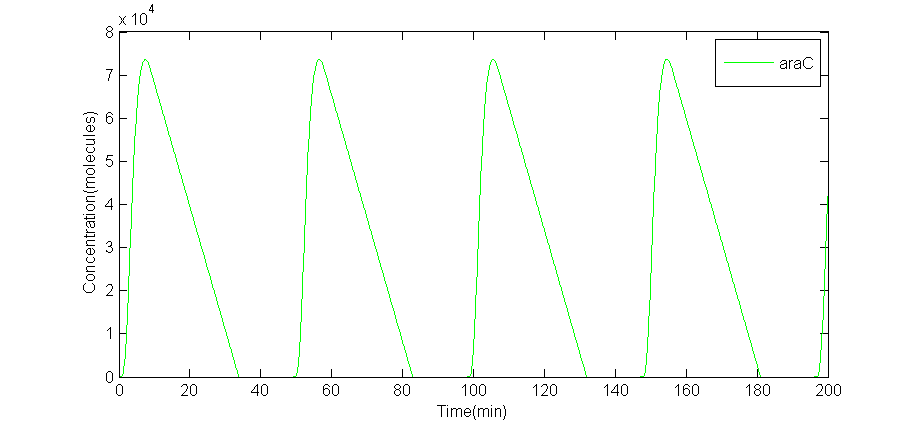(a) A numeric solve of AraC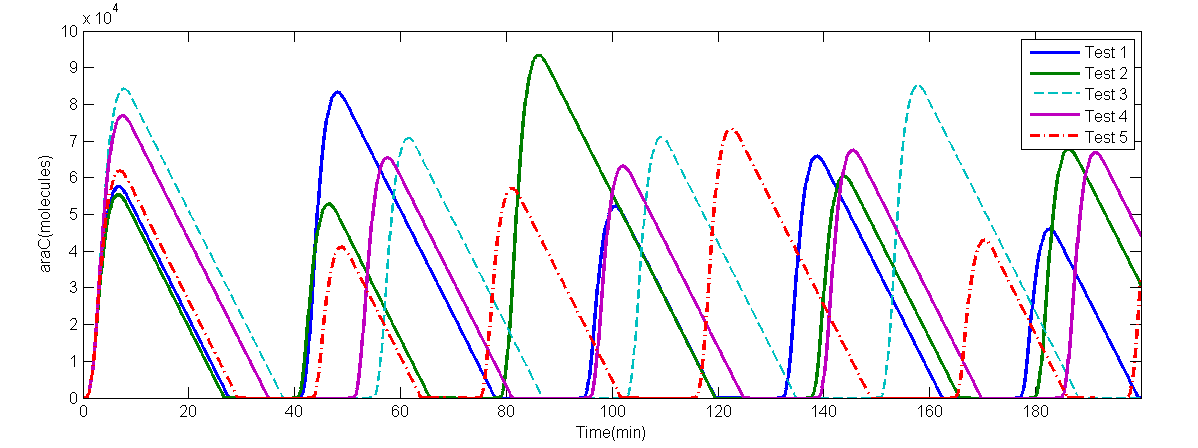(b) 5 random tests numeric solve of AraC

Fig 2.(a)A numeric solve of AraC when delay $\tau = \tau _1 = \tau _2 = 2$min, Arabinose concentration is 5%, IPTG concentration is 1mM, time interval is 0.1min. (b)numeric solve of AraC concentration versus time of 5 random tests, when Arabinose concentration is 0.7%, IPTG concentration is 10mM, and $\tau \sim N(2.0,0.3^2)$.

The period of this particular solve is 49.0minutes. The numeric solve of DDEs shows that the supposed oscillator is feasible. On the other hand, interval between every adjacent peak is different in a single random test, thus period is calculated by average intervals. Even so, the average period of each random test is different from each other: $T_1$= 43.95min, $T2$= 47.65min, $T_3$= 40.625min, $T_4$ = 39.375min, $T_5$ = 45.975min. Also, the amplitude of each curve is different. The random solve suggests that extern works should be concerned on forcing the period to be the same. However, due to limited time, we did not have such plan. To analyze whether it is stable against environment changes.

#### Stability of DDEs

Basing on the equations, since applying their Taylor series makes the equations formed in a linear one keeping the topology of the solution to the original equations[citation needed], the characteristic eqution can be presented: $$\left(\mu+\frac{\gamma}{(Ce+r)^2}\right)\left(\mu+k_{fr}+\frac{\gamma}{(Ce+r_{uf})^2}\right)(\mu + d_{a/r})E(\mu)=0$$ $$E(\mu) = \left(\mu+\lambda\frac{\gamma}{(Ce+a)^2}\right)(\mu+d_{a/r})\left(\mu+k_{fa}+\lambda\frac{\gamma}{(Ce+a_{uf})^2}\right)\\ -k_{fa}T_acopy_a(k_3E_1+k_4E_2+k_5E_3) = 0$$ $$\begin{cases} E_1 = \dfrac{\frac{2a}{a_0}e^{-2\mu \tau _1}\left(1+\frac{r^4}{r_0}e^{-4\mu \tau _2}\right)}{\left(1+\frac{r^4}{r_0}e^{-4 \mu \tau _2}+\frac{a^2}{a_0}e^{-2 \mu\tau _1}\right)^2}\\ E_2 = \dfrac{2ae^{-2 \mu\tau _1}\frac{r^2}{r_0}e^{-2 \mu\tau _2}}{\left(1+\frac{r^4}{r_0}e^{-4 \mu\tau _2}+\frac{a^2}{a_0}e^{-2\mu\tau _1}\right)^2}\\ E_3 = \dfrac{\frac{2a}{a_0}e^{-2\mu \tau _1}}{\left(1+\frac{r^4}{r_0}e^{-4 \mu\tau _2}+\frac{a^2}{a_0}e^{-2\mu\tau _1}\right)^2} \end{cases}$$ If there were at least one periodical solution, the equartion should have at least one imaginary root.
Denote$\begin{cases} c_1 = \lambda\frac{\gamma}{(Ce+a)^2}\\ c_2 = d_{a/r}\\ c_3 = k_{fa}+\lambda\frac{\gamma}{(Ce+a)^2}\\ c_4 = k_{fa}T_a(k_3E_1+k_4E_2+k_5E_3)copy_a \end{cases}$
Namely, $E(\mu) = (\mu+c_1)(\mu+c_2)(\mu+c_3)-c_4 = 0$ has imaginary root(s).
Set $\mu = iy$, $y\in R$,thus $iy(c_1c_2+c_2c_3+c_1c_3-y^2)-(c_1+c_2+c_3)y^2+c_1c_2c_3 = c_4$ is required for imaginary root(s).
Namely, $\begin{cases} y(c_1c_2+c_2c_3+c_1c_3-y^2) = Im(c_4) \\ -(c_1+c_2+c_3)y^2+c_1c_2c_3 = Re(c_4) \end{cases}$
Thus $y^5+(c_1^2+c_2^2)y^3+Im(c_4)y^2+(Re(c_4)(c_1+c_2)+c_1^2c_2^2)y-Im(c_4)c_1c_2=0$
Since $k_3 > k_4$,$k_3 > k_5$ , thus there is at least one positive real solution of the equation above. On the other hand, due to all the coefficients in this equation are positive, there is only one positive solution of this equation. In other words, there is one, and only one period for the oscillator. Detail is given below:
Apparently,$Re(c_4)>0$,$Im(c_4)>0$
Denote $G(y)=y^5+(c_1^2+c_2^2)y^3+Im(c_4)y^2+(Re(c_4)(c_1+c_2)+c_1^2c_1^2)y-Im(c_4)c_1c_2$
Thus, appararently,$\begin{cases} (c_1^2+c_2^2)>2 \\ Re(c_4)(c_1+c_2)+c_1^2c_2^2>0\\ -Im(c_4)c_1c_2<0 \\ \end{cases}$,since $c_1,c_2>0$
Thus $G(0)<0$,$G(+\infty)=+\infty$,thus there have to be at least one root.
Since $G'(y)=5y^4+3(c_1^2+c_2^2)y^2+2Im(y_4)y+(Re(c_4)(c_1+c_2)+c_1^2c_2^2)>0$,given $y>0$, the positive root is exclusive. In other words, the period exists and have unique existence.

#### Parameter Range and Sensitivity Analysis

To see how different concentration of both IPTG and Arabinose can affect the period of the oscillator , we solve the DDEs in various values of Arabinose and IPTG using R language. Both Arabinose and IPTG $\in [0,10]$, which is proved to be stable in the previous section. We use $\tau$ to replace both $\tau _1$ and $\tau _2$ since they are assumed to be the same.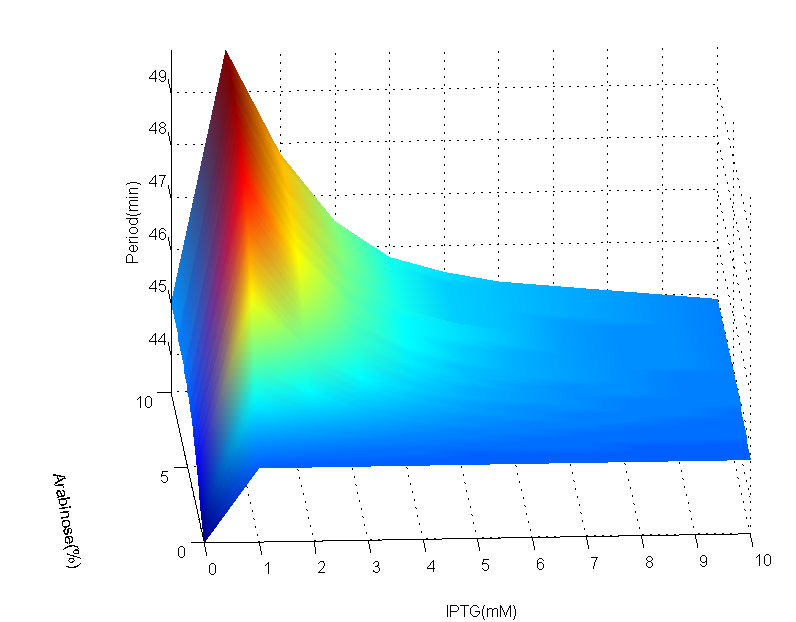(a)AraC period map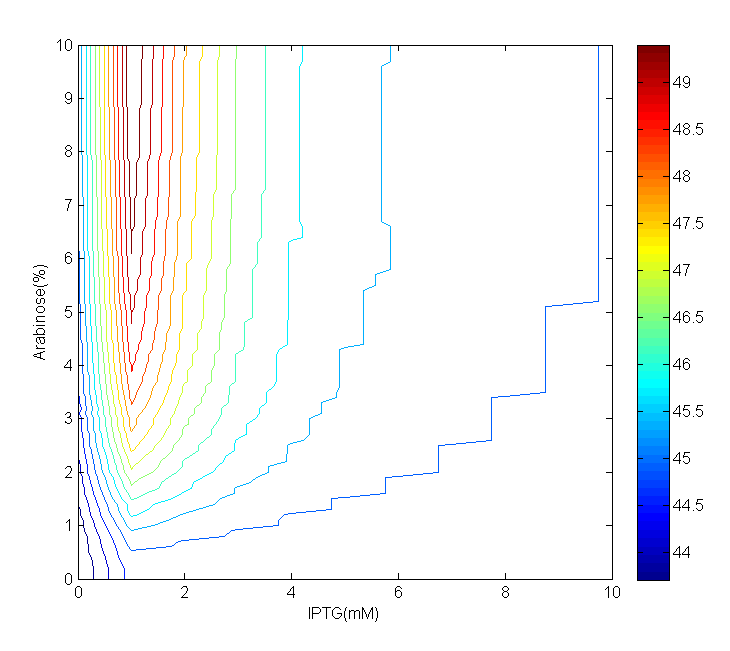(b)AraC period Contour

Fig 3.(a)AraC period map with IPTG from 0mM to 10mM, step is 1mM, Arabinose from 0% to 10%, step is 0.1%. (b)Contour of period concerning Arabinose and IPTG. In both figures, color shows the values of period.

The period map can be divided into two areas according to IPTG concentration: 'mountain'(0mM~5mM) and 'plain' (5mM~10mM). These areas can be more clearly seen in contour. The difference between largest and smallest period is approximately 6 minutes, which is insignificant comparing with the scale of period. In 'mountain' area, when IPTG concentration is fixed, the period increases alongside with Arabinose concentration; on the other hand, when Arabinose concentration is fixed, the period increases at first when IPTG concentration rises, then it decreases when IPTG concentration keep on rising. However, in 'plain' area, the period remain steady against either IPTG or Arabinose change.
Due to the computational expenses and our limited computing power, we set a rather large step. Though coarse, we can still grab the big picture of how IPTG and Arabinose can affect the period of the oscillator. We also examined a specific area in a small step size.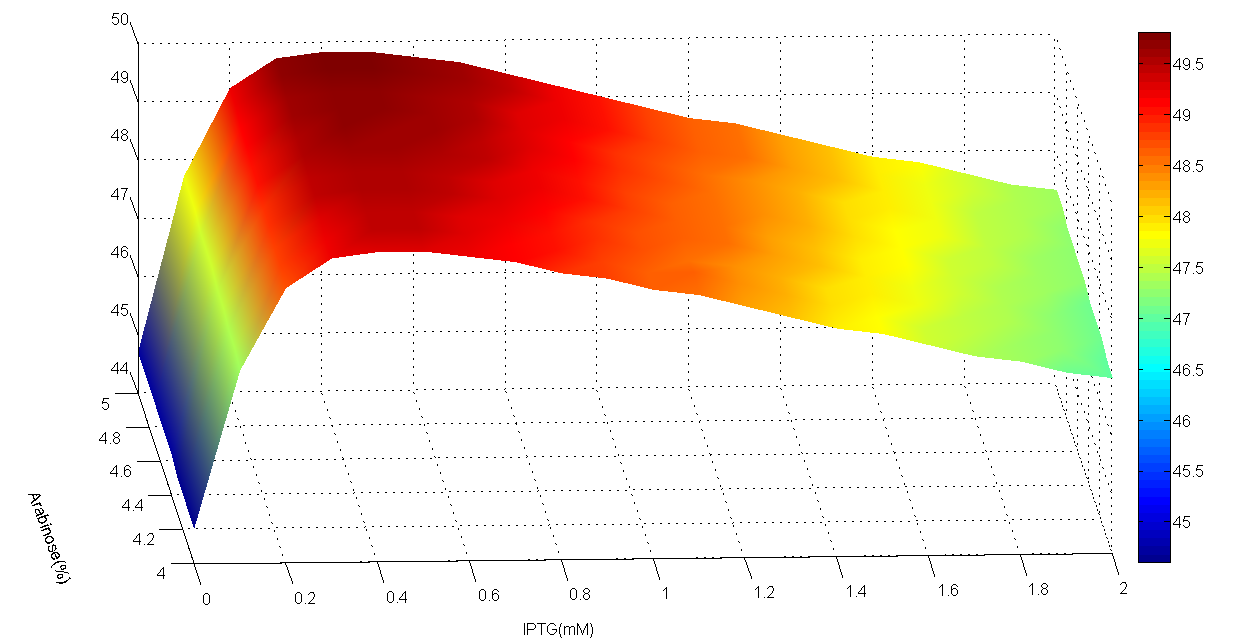Fig 4.AraC period of area whose IPTG is 0~2mM, step is 0.1mM and Arabinose is 4~5%, step is 0.1%. The edge is less sharp than the one above.

By examining specific area in a smaller step size, we found that surface of period is actually rather smooth, which suggests that a large step size does not limit its representativeness, since spline interpolation is used in plotting the discrete data and high accuracy is guaranteed by smoothness of the function.
Lastly, we examined the role of delay $\tau$ in the period of AraC.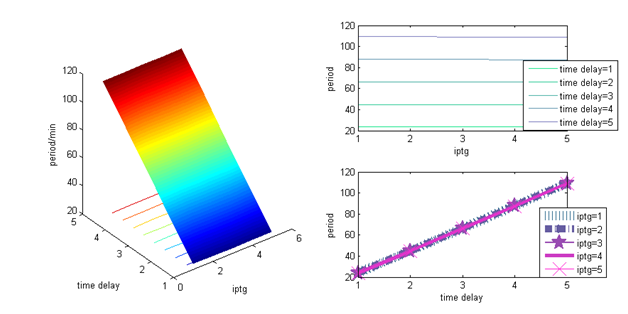Fig 5.AraC's period against delay $\tau$

In these figures, we could clearly see that AraC's period increases linearly as delay increases. To sum up, the range of period is rather limited while $\tau$ is fixed. In other word, it's rather stable against Arabinose and IPTG changes. On the other hand, we can see that $\tau$ has the biggest influence on AraC's period, which should be concerned firstly while adjusting the period of the oscillator. James D. Watson, 2007, Molecular Biology of the Gene - Sixth Edition, Cold Spring Harbor Laboratory Press, ISBN:9780805395921
Hendrickson W, Schleif R., 1985, A dimer of AraC protein contacts three adjacent major groove regions of the araI DNA site., Proc Natl Acad Sci U S A., 82(10):3129-33.
S Oehler, E R Eismann, H Krämer, and B Müller-Hill, 1990, The three operators of the lac operon cooperate in repression, Bembo. J., 9(4): 973–979.
Team:NTU-Singapore/Modelling/Parameter
Jarno Mäkelä et al., 2013, In vivo single-molecule kinetics of activation and subsequent activity of the arabinose promoter, Nucleic Acids Research, doi:10.1093/nar/gkt350
Jesse Stricker et al., 2008, Supplementary Information From A fast, robust and tunable synthetic gene oscillator, Nature 456, 516-519
Jack K. Hale, 1971, Functional Differential Equations, Lecture Notes in Mathematics Volume 183, pp 9-22